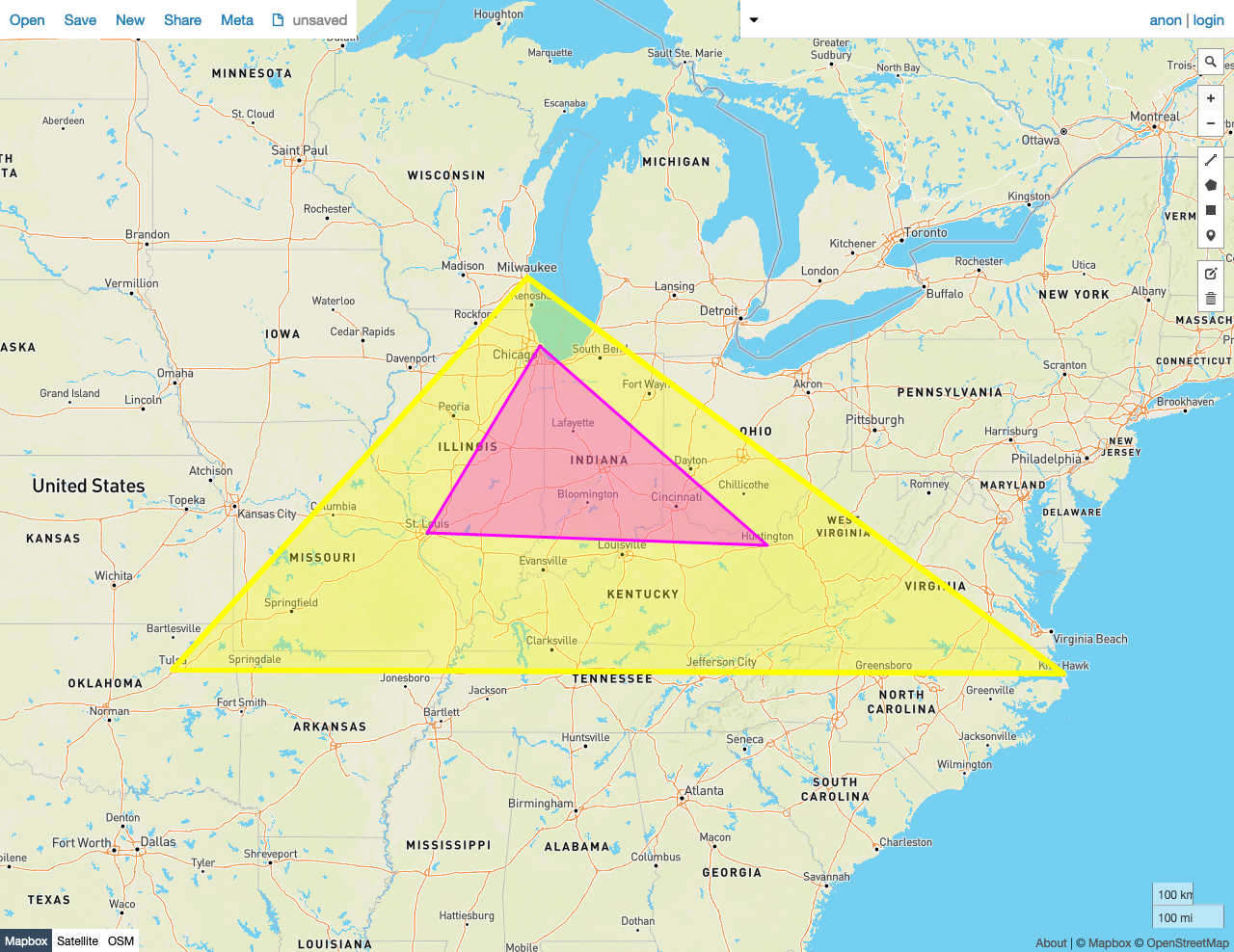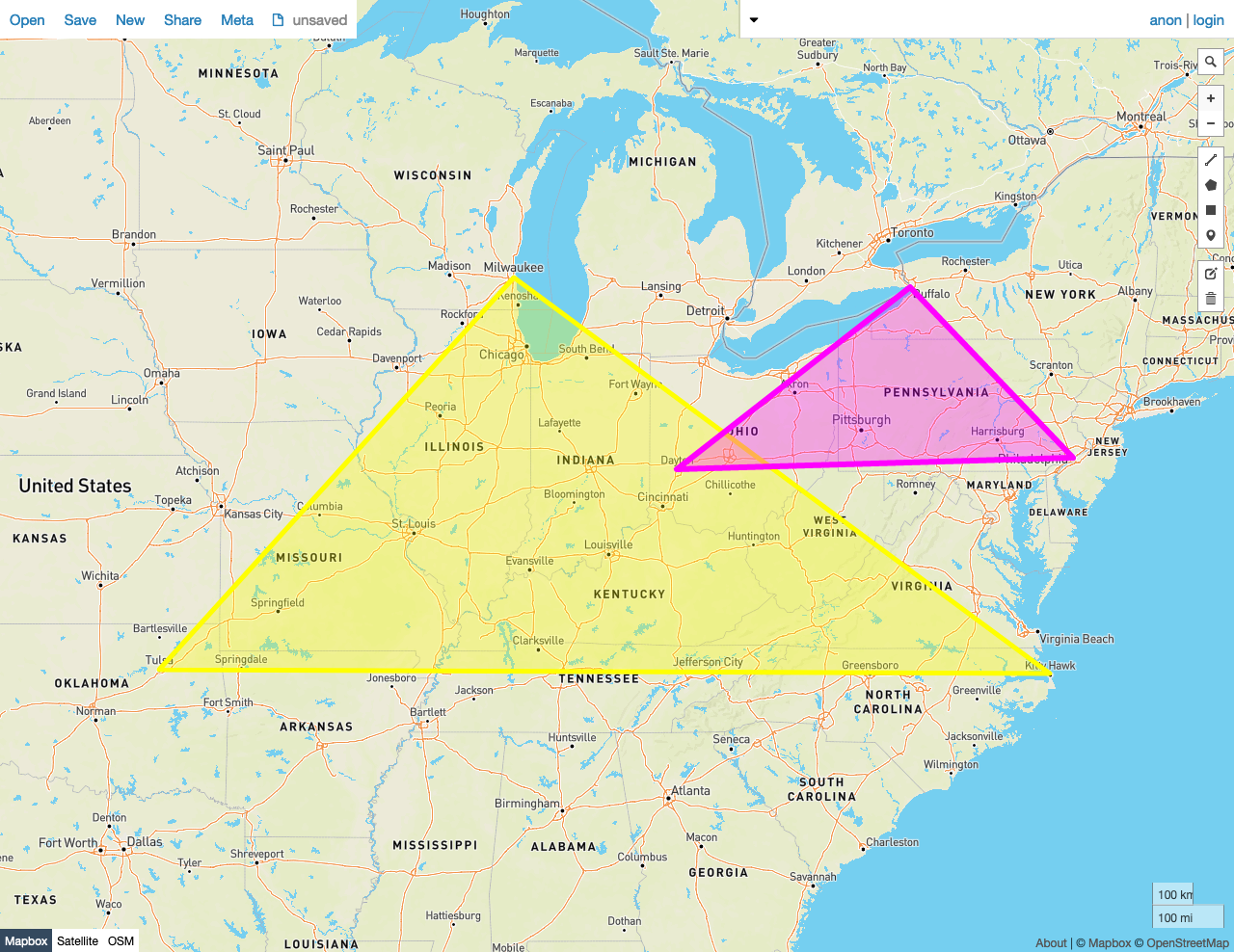Given two shapes A and B, predicate function `ST_Covers(A, B)` returns `true` if no point in B lies outside of shape A. Otherwise, it returns `false`.

In other words, shape A must completely cover every point in B.

This behavior is similar to `ST_Contains`, except that the criteria for `ST_Contains` are more exacting, and therefore some pairs of shapes will be accepted by this function that would be rejected by `ST_Contains`.

`ST_Covers` works on the following data types:

Note:

`ST_Covers` will attempt to use any available spatial index to speed up its operation. Use the prefixed variant `_ST_Covers` if you do not want any spatial indexes to be used.

Note:

This function is the inverse of `ST_CoveredBy`.

## Examples

Note:

The screenshots in these examples were generated using geojson.io, but they are designed to showcase the shapes, not the map. Representing `GEOMETRY` data in GeoJSON can lead to unexpected results if using geometries with SRIDs other than 4326 (as shown below).

### True

In this example, `ST_Covers` returns `true` because:

• No Point in the smaller Polygon B lies outside of the larger Polygon A.
``````SELECT ST_Covers(st_geomfromtext('SRID=4326;POLYGON((-87.906471 43.038902, -95.992775 36.153980, -75.704722 36.076944, -87.906471 43.038902))'), st_geomfromtext('SRID=4326;POLYGON((-87.623177 41.881832, -90.199402 38.627003, -82.446732 38.413651, -87.623177 41.881832))'));
``````
``````   st_covers
---------------
true

(1 row)
``````### False

In this example, `ST_Covers` returns `false` because:

• Many Points in the smaller Polygon B lie outside of the larger Polygon A.
``````SELECT ST_Covers(st_geomfromtext('SRID=4326;POLYGON((-87.906471 43.038902, -95.992775 36.153980, -75.704722 36.076944, -87.906471 43.038902))'), st_geomfromtext('SRID=4326;POLYGON((-84.191605 39.758949, -75.165222 39.952583, -78.878738 42.880230, -84.191605 39.758949))'));
``````
``````   st_covers
---------------
false
(1 row)
``````